# Tuning for Systematic Trading: Talk 3: Training, Tuning, and Metric Strategy

May. 19, 2020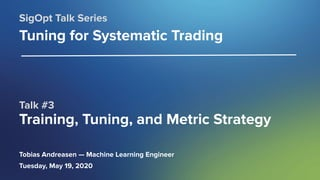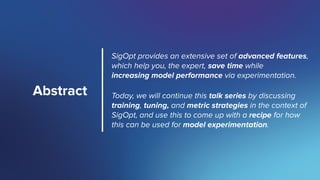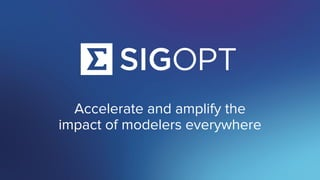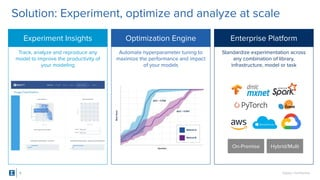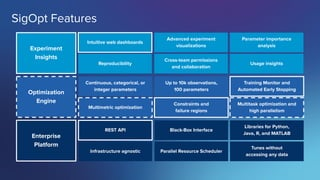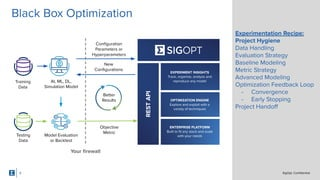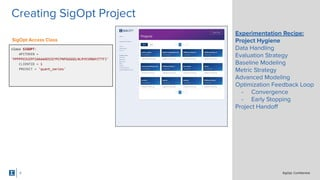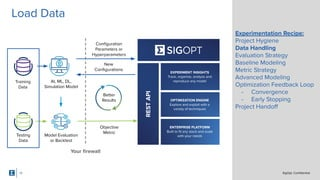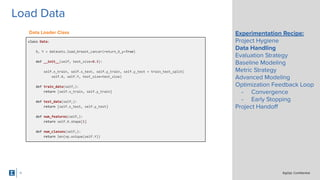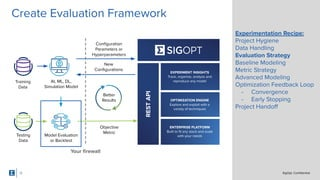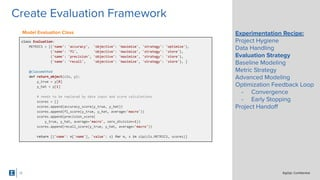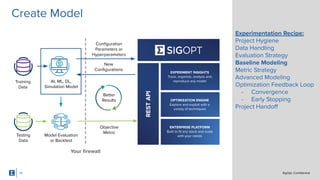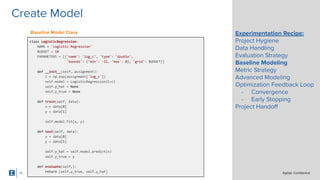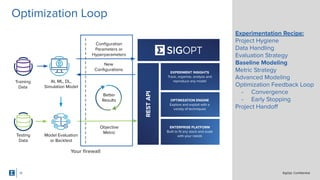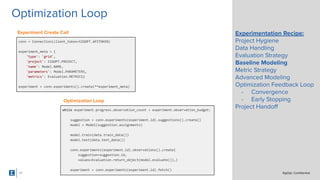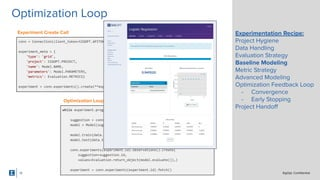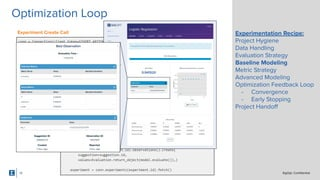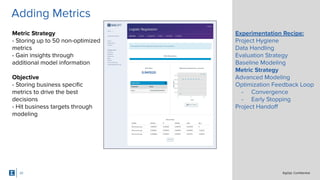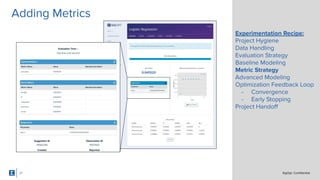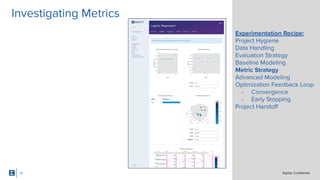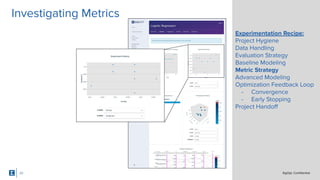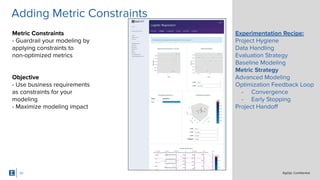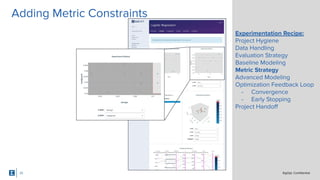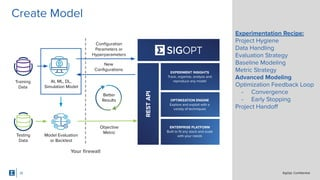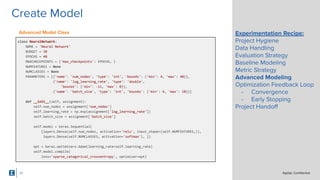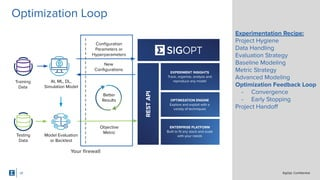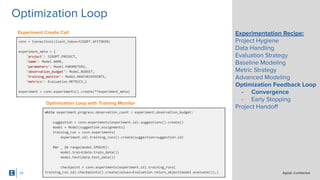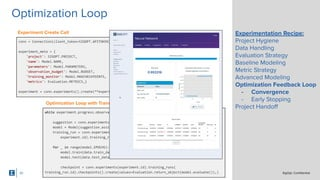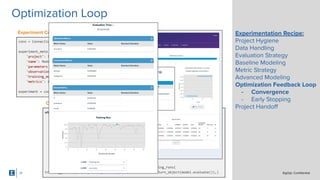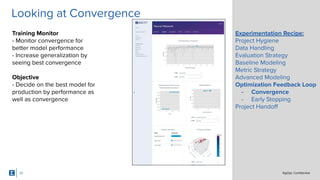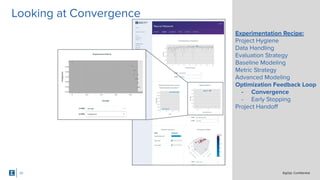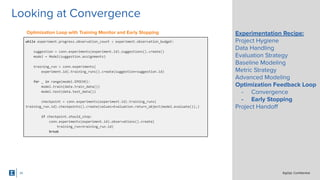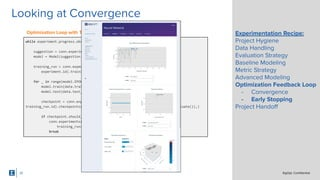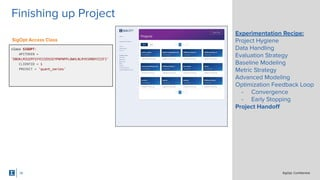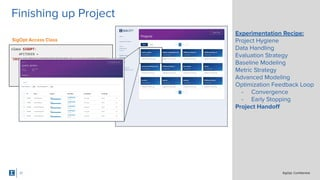1 of 38

### Tuning for Systematic Trading: Talk 3: Training, Tuning, and Metric Strategy

1. SigOpt. Conﬁdential. Talk #3 Training, Tuning, and Metric Strategy SigOpt Talk Series Tuning for Systematic Trading Tobias Andreasen — Machine Learning Engineer Tuesday, May 19, 2020
2. SigOpt. Conﬁdential. Abstract SigOpt provides an extensive set of advanced features, which help you, the expert, save time while increasing model performance via experimentation. Today, we will continue this talk series by discussing training, tuning, and metric strategies in the context of SigOpt, and use this to come up with a recipe for how this can be used for model experimentation.
3. SigOpt. Conﬁdential. Overview of SigOpt
4. SigOpt. Conﬁdential. Accelerate and amplify the impact of modelers everywhere
5. SigOpt. Conﬁdential. Experiment Insights Optimization Engine Track, analyze and reproduce any model to improve the productivity of your modeling Enterprise Platform Automate hyperparameter tuning to maximize the performance and impact of your models Standardize experimentation across any combination of library, infrastructure, model or task On-Premise Hybrid/Multi Solution: Experiment, optimize and analyze at scale 5
6. SigOpt. Conﬁdential. SigOpt Features Enterprise Platform Optimization Engine Experiment Insights Reproducibility Intuitive web dashboards Cross-team permissions and collaboration Advanced experiment visualizations Usage insights Parameter importance analysis Multimetric optimization Continuous, categorical, or integer parameters Constraints and failure regions Up to 10k observations, 100 parameters Multitask optimization and high parallelism Training Monitor and Automated Early Stopping Infrastructure agnostic REST API Parallel Resource Scheduler Black-Box Interface Tunes without accessing any data Libraries for Python, Java, R, and MATLAB
7. SigOpt. Conﬁdential. Experimentation Recipe
8. SigOpt. Conﬁdential.SigOpt. Conﬁdential. Key Use Cases Black Box Optimization Your ﬁrewall Training Data AI, ML, DL, Simulation Model Model Evaluation or Backtest Testing Data New Conﬁgurations Objective Metric Better Results EXPERIMENT INSIGHTS Track, organize, analyze and reproduce any model ENTERPRISE PLATFORM Built to ﬁt any stack and scale with your needs OPTIMIZATION ENGINE Explore and exploit with a variety of techniques RESTAPI Conﬁguration Parameters or Hyperparameters Experimentation Recipe: Project Hygiene Data Handling Evaluation Strategy Baseline Modeling Metric Strategy Advanced Modeling Optimization Feedback Loop - Convergence - Early Stopping Project Handoﬀ 8
9. SigOpt. Conﬁdential.SigOpt. Conﬁdential. Key Use Cases 9 Experimentation Recipe: Project Hygiene Data Handling Evaluation Strategy Baseline Modeling Metric Strategy Advanced Modeling Optimization Feedback Loop - Convergence - Early Stopping Project Handoﬀ class SIGOPT: APITOKEN = 'PPPPPOJUZPFSAAAAADSSEYPCPNPGGGGGLNLRYKSRBWYZTTFI' CLIENTID = 1 PROJECT = 'quant_series' Creating SigOpt Project SigOpt Access Class
10. SigOpt. Conﬁdential.SigOpt. Conﬁdential. Key Use Cases Your ﬁrewall Training Data AI, ML, DL, Simulation Model Model Evaluation or Backtest Testing Data New Conﬁgurations Objective Metric Better Results EXPERIMENT INSIGHTS Track, organize, analyze and reproduce any model ENTERPRISE PLATFORM Built to ﬁt any stack and scale with your needs OPTIMIZATION ENGINE Explore and exploit with a variety of techniques RESTAPI Conﬁguration Parameters or Hyperparameters 10 Experimentation Recipe: Project Hygiene Data Handling Evaluation Strategy Baseline Modeling Metric Strategy Advanced Modeling Optimization Feedback Loop - Convergence - Early Stopping Project Handoﬀ Load Data
11. SigOpt. Conﬁdential.SigOpt. Conﬁdential. Key Use Cases 11 Experimentation Recipe: Project Hygiene Data Handling Evaluation Strategy Baseline Modeling Metric Strategy Advanced Modeling Optimization Feedback Loop - Convergence - Early Stopping Project Handoﬀ class Data: X, Y = datasets.load_breast_cancer(return_X_y=True) def __init__(self, test_size=0.3): self.x_train, self.x_test, self.y_train, self.y_test = train_test_split( self.X, self.Y, test_size=test_size) def train_data(self,): return [self.x_train, self.y_train] def test_data(self,): return [self.x_test, self.y_test] def num_features(self,): return self.X.shape def num_classes(self,): return len(np.unique(self.Y)) Load Data Data Loader Class
12. SigOpt. Conﬁdential.SigOpt. Conﬁdential. Key Use Cases Your ﬁrewall Training Data AI, ML, DL, Simulation Model Model Evaluation or Backtest Testing Data New Conﬁgurations Objective Metric Better Results EXPERIMENT INSIGHTS Track, organize, analyze and reproduce any model ENTERPRISE PLATFORM Built to ﬁt any stack and scale with your needs OPTIMIZATION ENGINE Explore and exploit with a variety of techniques RESTAPI Conﬁguration Parameters or Hyperparameters 12 Experimentation Recipe: Project Hygiene Data Handling Evaluation Strategy Baseline Modeling Metric Strategy Advanced Modeling Optimization Feedback Loop - Convergence - Early Stopping Project Handoﬀ Create Evaluation Framework
13. SigOpt. Conﬁdential.SigOpt. Conﬁdential. Key Use Cases 13 Experimentation Recipe: Project Hygiene Data Handling Evaluation Strategy Baseline Modeling Metric Strategy Advanced Modeling Optimization Feedback Loop - Convergence - Early Stopping Project Handoﬀ class Evaluation: METRICS = [{'name': 'accuracy', 'objective': 'maximize', 'strategy': 'optimize'}, {'name': 'f1', 'objective': 'maximize', 'strategy': 'store'}, {'name': 'precision', 'objective': 'maximize', 'strategy': 'store'}, {'name': 'recall', 'objective': 'maximize', 'strategy': 'store'}, ] @classmethod def return_object(cls, y): y_true = y y_hat = y # needs to be replaced by data input and score calculations scores = [] scores.append(accuracy_score(y_true, y_hat)) scores.append(f1_score(y_true, y_hat, average='macro')) scores.append(precision_score( y_true, y_hat, average='macro', zero_division=1)) scores.append(recall_score(y_true, y_hat, average='macro')) return [{'name': m['name'], 'value': s} for m, s in zip(cls.METRICS, scores)] Create Evaluation Framework Model Evaluation Class
14. SigOpt. Conﬁdential.SigOpt. Conﬁdential. Key Use Cases Your ﬁrewall Training Data AI, ML, DL, Simulation Model Model Evaluation or Backtest Testing Data New Conﬁgurations Objective Metric Better Results EXPERIMENT INSIGHTS Track, organize, analyze and reproduce any model ENTERPRISE PLATFORM Built to ﬁt any stack and scale with your needs OPTIMIZATION ENGINE Explore and exploit with a variety of techniques RESTAPI Conﬁguration Parameters or Hyperparameters 14 Experimentation Recipe: Project Hygiene Data Handling Evaluation Strategy Baseline Modeling Metric Strategy Advanced Modeling Optimization Feedback Loop - Convergence - Early Stopping Project Handoﬀ Create Model
15. SigOpt. Conﬁdential.SigOpt. Conﬁdential. Key Use Cases 15 Experimentation Recipe: Project Hygiene Data Handling Evaluation Strategy Baseline Modeling Metric Strategy Advanced Modeling Optimization Feedback Loop - Convergence - Early Stopping Project Handoﬀ class LogisticRegression: NAME = 'Logistic Regression' BUDGET = 10 PARAMETERS = [{'name': 'log_c', 'type': 'double', 'bounds': {'min': -11, 'max': 0}, 'grid': BUDGET}] def __init__(self, assignment): c = np.exp(assignment['log_c']) self.model = LogisticRegression(C=c) self.y_hat = None self.y_true = None def train(self, data): x = data y = data self.model.fit(x, y) def test(self, data): x = data y = data self.y_hat = self.model.predict(x) self.y_true = y def evaluate(self,): return [self.y_true, self.y_hat] Create Model Baseline Model Class
16. SigOpt. Conﬁdential.SigOpt. Conﬁdential. Key Use Cases Your ﬁrewall Training Data AI, ML, DL, Simulation Model Model Evaluation or Backtest Testing Data New Conﬁgurations Objective Metric Better Results EXPERIMENT INSIGHTS Track, organize, analyze and reproduce any model ENTERPRISE PLATFORM Built to ﬁt any stack and scale with your needs OPTIMIZATION ENGINE Explore and exploit with a variety of techniques RESTAPI Conﬁguration Parameters or Hyperparameters 16 Experimentation Recipe: Project Hygiene Data Handling Evaluation Strategy Baseline Modeling Metric Strategy Advanced Modeling Optimization Feedback Loop - Convergence - Early Stopping Project Handoﬀ Optimization Loop
17. SigOpt. Conﬁdential.SigOpt. Conﬁdential. Key Use Cases 17 Experimentation Recipe: Project Hygiene Data Handling Evaluation Strategy Baseline Modeling Metric Strategy Advanced Modeling Optimization Feedback Loop - Convergence - Early Stopping Project Handoﬀ conn = Connection(client_token=SIGOPT.APITOKEN) experiment_meta = { 'type': 'grid', 'project': SIGOPT.PROJECT, 'name': Model.NAME, 'parameters': Model.PARAMETERS, 'metrics': Evaluation.METRICS} experiment = conn.experiments().create(**experiment_meta) while experiment.progress.observation_count < experiment.observation_budget: suggestion = conn.experiments(experiment.id).suggestions().create() model = Model(suggestion.assignments) model.train(data.train_data()) model.test(data.test_data()) conn.experiments(experiment.id).observations().create( suggestion=suggestion.id, values=Evaluation.return_object(model.evaluate()),) experiment = conn.experiments(experiment.id).fetch() Optimization Loop Experiment Create Call Optimization Loop
18. SigOpt. Conﬁdential.SigOpt. Conﬁdential. Key Use Cases 18 Experimentation Recipe: Project Hygiene Data Handling Evaluation Strategy Baseline Modeling Metric Strategy Advanced Modeling Optimization Feedback Loop - Convergence - Early Stopping Project Handoﬀ conn = Connection(client_token=SIGOPT.APITOKEN) experiment_meta = { 'type': 'grid', 'project': SIGOPT.PROJECT, 'name': Model.NAME, 'parameters': Model.PARAMETERS, 'metrics': Evaluation.METRICS} experiment = conn.experiments().create(**experiment_meta) while experiment.progress.observation_count < experiment.observation_budget: suggestion = conn.experiments(experiment.id).suggestions().create() model = Model(suggestion.assignments) model.train(data.train_data()) model.test(data.test_data()) conn.experiments(experiment.id).observations().create( suggestion=suggestion.id, values=Evaluation.return_object(model.evaluate()),) experiment = conn.experiments(experiment.id).fetch() Optimization Loop Experiment Create Call Optimization Loop
19. SigOpt. Conﬁdential.SigOpt. Conﬁdential. Key Use Cases 19 Experimentation Recipe: Project Hygiene Data Handling Evaluation Strategy Baseline Modeling Metric Strategy Advanced Modeling Optimization Feedback Loop - Convergence - Early Stopping Project Handoﬀ conn = Connection(client_token=SIGOPT.APITOKEN) experiment_meta = { 'type': 'grid', 'project': SIGOPT.PROJECT, 'name': Model.NAME, 'parameters': Model.PARAMETERS, 'metrics': Evaluation.METRICS} experiment = conn.experiments().create(**experiment_meta) while experiment.progress.observation_count < experiment.observation_budget: suggestion = conn.experiments(experiment.id).suggestions().create() model = Model(suggestion.assignments) model.train(data.train_data()) model.test(data.test_data()) conn.experiments(experiment.id).observations().create( suggestion=suggestion.id, values=Evaluation.return_object(model.evaluate()),) experiment = conn.experiments(experiment.id).fetch() Optimization Loop Experiment Create Call Optimization Loop
20. SigOpt. Conﬁdential.SigOpt. Conﬁdential. Key Use Cases 20 Experimentation Recipe: Project Hygiene Data Handling Evaluation Strategy Baseline Modeling Metric Strategy Advanced Modeling Optimization Feedback Loop - Convergence - Early Stopping Project Handoﬀ Metric Strategy - Storing up to 50 non-optimized metrics - Gain insights through additional model information Objective - Storing business speciﬁc metrics to drive the best decisions - Hit business targets through modeling Adding Metrics
21. SigOpt. Conﬁdential.SigOpt. Conﬁdential. Key Use Cases 21 Experimentation Recipe: Project Hygiene Data Handling Evaluation Strategy Baseline Modeling Metric Strategy Advanced Modeling Optimization Feedback Loop - Convergence - Early Stopping Project Handoﬀ Adding Metrics
22. SigOpt. Conﬁdential.SigOpt. Conﬁdential. Key Use Cases 22 Experimentation Recipe: Project Hygiene Data Handling Evaluation Strategy Baseline Modeling Metric Strategy Advanced Modeling Optimization Feedback Loop - Convergence - Early Stopping Project Handoﬀ Investigating Metrics
23. SigOpt. Conﬁdential.SigOpt. Conﬁdential. Key Use Cases 23 Experimentation Recipe: Project Hygiene Data Handling Evaluation Strategy Baseline Modeling Metric Strategy Advanced Modeling Optimization Feedback Loop - Convergence - Early Stopping Project Handoﬀ Investigating Metrics
24. SigOpt. Conﬁdential.SigOpt. Conﬁdential. Key Use Cases 24 Experimentation Recipe: Project Hygiene Data Handling Evaluation Strategy Baseline Modeling Metric Strategy Advanced Modeling Optimization Feedback Loop - Convergence - Early Stopping Project Handoﬀ Metric Constraints - Guardrail your modeling by applying constraints to non-optimized metrics Objective - Use business requirements as constraints for your modeling - Maximize modeling impact Adding Metric Constraints
25. SigOpt. Conﬁdential.SigOpt. Conﬁdential. Key Use Cases 25 Experimentation Recipe: Project Hygiene Data Handling Evaluation Strategy Baseline Modeling Metric Strategy Advanced Modeling Optimization Feedback Loop - Convergence - Early Stopping Project Handoﬀ Adding Metric Constraints
26. SigOpt. Conﬁdential.SigOpt. Conﬁdential. Key Use Cases Your ﬁrewall Training Data AI, ML, DL, Simulation Model Model Evaluation or Backtest Testing Data New Conﬁgurations Objective Metric Better Results EXPERIMENT INSIGHTS Track, organize, analyze and reproduce any model ENTERPRISE PLATFORM Built to ﬁt any stack and scale with your needs OPTIMIZATION ENGINE Explore and exploit with a variety of techniques RESTAPI Conﬁguration Parameters or Hyperparameters 26 Experimentation Recipe: Project Hygiene Data Handling Evaluation Strategy Baseline Modeling Metric Strategy Advanced Modeling Optimization Feedback Loop - Convergence - Early Stopping Project Handoﬀ Create Model
27. SigOpt. Conﬁdential.SigOpt. Conﬁdential. Key Use Cases 27 Experimentation Recipe: Project Hygiene Data Handling Evaluation Strategy Baseline Modeling Metric Strategy Advanced Modeling Optimization Feedback Loop - Convergence - Early Stopping Project Handoﬀ class NeuralNetwork: NAME = 'Neural Network' BUDGET = 30 EPOCHS = 40 MAXCHECKPOINTS = {'max_checkpoints': EPOCHS, } NUMFEATURES = None NUMCLASSES = None PARAMETERS = [{'name': 'num_nodes', 'type': 'int', 'bounds': {'min': 4, 'max': 40}}, {'name': 'log_learning_rate', 'type': 'double', 'bounds': {'min': -11, 'max': 0}}, {'name': 'batch_size', 'type': 'int', 'bounds': {'min': 4, 'max': 10}}] def __init__(self, assignment): self.num_nodes = assignment['num_nodes'] self.learning_rate = np.exp(assignment['log_learning_rate']) self.batch_size = assignment['batch_size'] self.model = keras.Sequential( [layers.Dense(self.num_nodes, activation='relu', input_shape=(self.NUMFEATURES,)), layers.Dense(self.NUMCLASSES, activation='softmax'), ]) opt = keras.optimizers.Adam(learning_rate=self.learning_rate) self.model.compile( loss='sparse_categorical_crossentropy', optimizer=opt) Create Model Advanced Model Class
28. SigOpt. Conﬁdential.SigOpt. Conﬁdential. Key Use Cases Your ﬁrewall Training Data AI, ML, DL, Simulation Model Model Evaluation or Backtest Testing Data New Conﬁgurations Objective Metric Better Results EXPERIMENT INSIGHTS Track, organize, analyze and reproduce any model ENTERPRISE PLATFORM Built to ﬁt any stack and scale with your needs OPTIMIZATION ENGINE Explore and exploit with a variety of techniques RESTAPI Conﬁguration Parameters or Hyperparameters 28 Experimentation Recipe: Project Hygiene Data Handling Evaluation Strategy Baseline Modeling Metric Strategy Advanced Modeling Optimization Feedback Loop - Convergence - Early Stopping Project Handoﬀ Optimization Loop
29. SigOpt. Conﬁdential.SigOpt. Conﬁdential. Key Use Cases 29 Experimentation Recipe: Project Hygiene Data Handling Evaluation Strategy Baseline Modeling Metric Strategy Advanced Modeling Optimization Feedback Loop - Convergence - Early Stopping Project Handoﬀ conn = Connection(client_token=SIGOPT.APITOKEN) experiment_meta = { 'project': SIGOPT.PROJECT, 'name': Model.NAME, 'parameters': Model.PARAMETERS, 'observation_budget': Model.BUDGET, 'training_monitor': Model.MAXCHECKPOINTS, 'metrics': Evaluation.METRICS,} experiment = conn.experiments().create(**experiment_meta) while experiment.progress.observation_count < experiment.observation_budget: suggestion = conn.experiments(experiment.id).suggestions().create() model = Model(suggestion.assignments) training_run = conn.experiments( experiment.id).training_runs().create(suggestion=suggestion.id) for _ in range(model.EPOCHS): model.train(data.train_data()) model.test(data.test_data()) checkpoint = conn.experiments(experiment.id).training_runs( training_run.id).checkpoints().create(values=Evaluation.return_object(model.evaluate()),) Optimization Loop Experiment Create Call Optimization Loop with Training Monitor
30. SigOpt. Conﬁdential.SigOpt. Conﬁdential. Key Use Cases 30 Experimentation Recipe: Project Hygiene Data Handling Evaluation Strategy Baseline Modeling Metric Strategy Advanced Modeling Optimization Feedback Loop - Convergence - Early Stopping Project Handoﬀ conn = Connection(client_token=SIGOPT.APITOKEN) experiment_meta = { 'project': SIGOPT.PROJECT, 'name': Model.NAME, 'parameters': Model.PARAMETERS, 'observation_budget': Model.BUDGET, 'training_monitor': Model.MAXCHECKPOINTS, 'metrics': Evaluation.METRICS,} experiment = conn.experiments().create(**experiment_meta) while experiment.progress.observation_count < experiment.observation_budget: suggestion = conn.experiments(experiment.id).suggestions().create() model = Model(suggestion.assignments) training_run = conn.experiments( experiment.id).training_runs().create(suggestion=suggestion.id) for _ in range(model.EPOCHS): model.train(data.train_data()) model.test(data.test_data()) checkpoint = conn.experiments(experiment.id).training_runs( training_run.id).checkpoints().create(values=Evaluation.return_object(model.evaluate()),) Optimization Loop Experiment Create Call Optimization Loop with Training Monitor
31. SigOpt. Conﬁdential.SigOpt. Conﬁdential. Key Use Cases Optimization Loop with Training Monitor 31 Experimentation Recipe: Project Hygiene Data Handling Evaluation Strategy Baseline Modeling Metric Strategy Advanced Modeling Optimization Feedback Loop - Convergence - Early Stopping Project Handoﬀ conn = Connection(client_token=SIGOPT.APITOKEN) experiment_meta = { 'project': SIGOPT.PROJECT, 'name': Model.NAME, 'parameters': Model.PARAMETERS, 'observation_budget': Model.BUDGET, 'training_monitor': Model.MAXCHECKPOINTS, 'metrics': Evaluation.METRICS,} experiment = conn.experiments().create(**experiment_meta) while experiment.progress.observation_count < experiment.observation_budget: suggestion = conn.experiments(experiment.id).suggestions().create() model = Model(suggestion.assignments) training_run = conn.experiments( experiment.id).training_runs().create(suggestion=suggestion.id) for _ in range(model.EPOCHS): model.train(data.train_data()) model.test(data.test_data()) checkpoint = conn.experiments(experiment.id).training_runs( training_run.id).checkpoints().create(values=Evaluation.return_object(model.evaluate()),) Optimization Loop Experiment Create Call
32. SigOpt. Conﬁdential.SigOpt. Conﬁdential. Key Use Cases 32 Experimentation Recipe: Project Hygiene Data Handling Evaluation Strategy Baseline Modeling Metric Strategy Advanced Modeling Optimization Feedback Loop - Convergence - Early Stopping Project Handoﬀ Training Monitor - Monitor convergence for better model performance - Increase generalization by seeing best convergence Objective - Decide on the best model for production by performance as well as convergence Looking at Convergence
33. SigOpt. Conﬁdential.SigOpt. Conﬁdential. Key Use Cases 33 Experimentation Recipe: Project Hygiene Data Handling Evaluation Strategy Baseline Modeling Metric Strategy Advanced Modeling Optimization Feedback Loop - Convergence - Early Stopping Project Handoﬀ Looking at Convergence
34. SigOpt. Conﬁdential.SigOpt. Conﬁdential. Key Use Cases 34 Experimentation Recipe: Project Hygiene Data Handling Evaluation Strategy Baseline Modeling Metric Strategy Advanced Modeling Optimization Feedback Loop - Convergence - Early Stopping Project Handoﬀ while experiment.progress.observation_count < experiment.observation_budget: suggestion = conn.experiments(experiment.id).suggestions().create() model = Model(suggestion.assignments) training_run = conn.experiments( experiment.id).training_runs().create(suggestion=suggestion.id) for _ in range(model.EPOCHS): model.train(data.train_data()) model.test(data.test_data()) checkpoint = conn.experiments(experiment.id).training_runs( training_run.id).checkpoints().create(values=Evaluation.return_object(model.evaluate()),) if checkpoint.should_stop: conn.experiments(experiment.id).observations().create( training_run=training_run.id) break Looking at Convergence Optimization Loop with Training Monitor and Early Stopping
35. SigOpt. Conﬁdential.SigOpt. Conﬁdential. Key Use Cases 35 Experimentation Recipe: Project Hygiene Data Handling Evaluation Strategy Baseline Modeling Metric Strategy Advanced Modeling Optimization Feedback Loop - Convergence - Early Stopping Project Handoﬀ while experiment.progress.observation_count < experiment.observation_budget: suggestion = conn.experiments(experiment.id).suggestions().create() model = Model(suggestion.assignments) training_run = conn.experiments( experiment.id).training_runs().create(suggestion=suggestion.id) for _ in range(model.EPOCHS): model.train(data.train_data()) model.test(data.test_data()) checkpoint = conn.experiments(experiment.id).training_runs( training_run.id).checkpoints().create(values=Evaluation.return_object(model.evaluate()),) if checkpoint.should_stop: conn.experiments(experiment.id).observations().create( training_run=training_run.id) break Looking at Convergence Optimization Loop with Training Monitor and Early Stopping
36. SigOpt. Conﬁdential.SigOpt. Conﬁdential. Key Use Cases 36 Experimentation Recipe: Project Hygiene Data Handling Evaluation Strategy Baseline Modeling Metric Strategy Advanced Modeling Optimization Feedback Loop - Convergence - Early Stopping Project Handoﬀ class SIGOPT: APITOKEN = 'DBOKLMJUZPFSYYCCEDSSEYPNPNPPLOWHLNLRYKSRBDYZIIFI' CLIENTID = 1 PROJECT = 'quant_series' Finishing up Project SigOpt Access Class
37. SigOpt. Conﬁdential.SigOpt. Conﬁdential. Key Use Casesclass SIGOPT: APITOKEN = 'DBOKLMJUZPFSYYCCEDSSEYPNPNPPLOWHLNLRYKSRBDYZIIFI' CLIENTID = 1 PROJECT = 'quant_series' 37 Experimentation Recipe: Project Hygiene Data Handling Evaluation Strategy Baseline Modeling Metric Strategy Advanced Modeling Optimization Feedback Loop - Convergence - Early Stopping Project Handoﬀ Finishing up Project SigOpt Access Class
38. SigOpt. Conﬁdential. Tobias Andreasen | tobias@sigopt.com For more information visit: https://sigopt.com/research/ Questions?